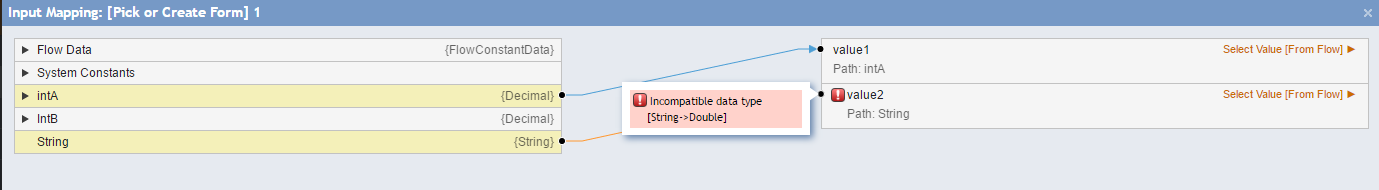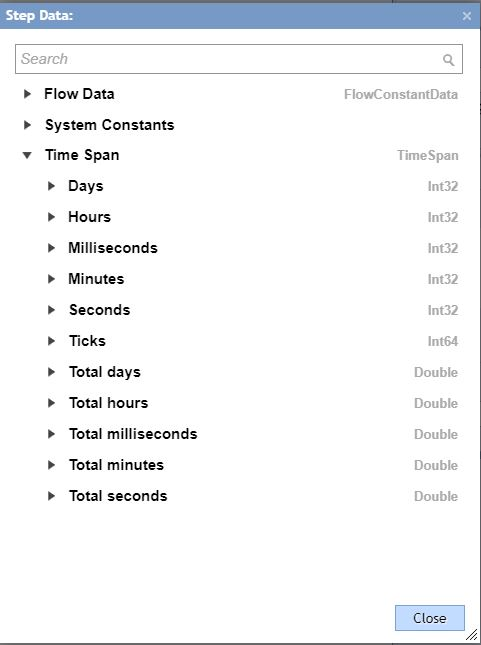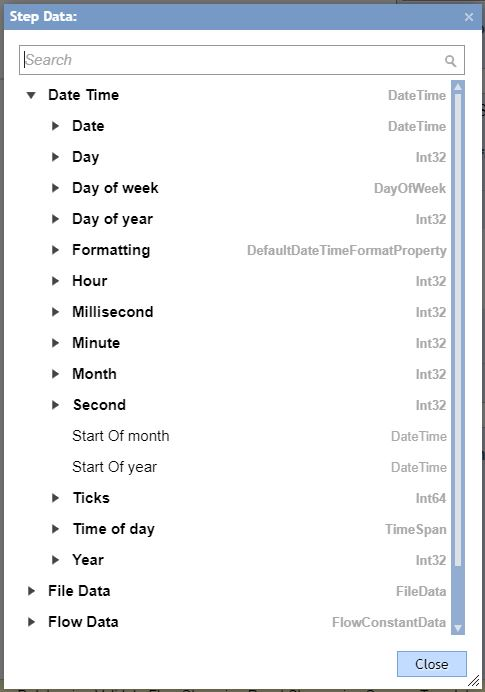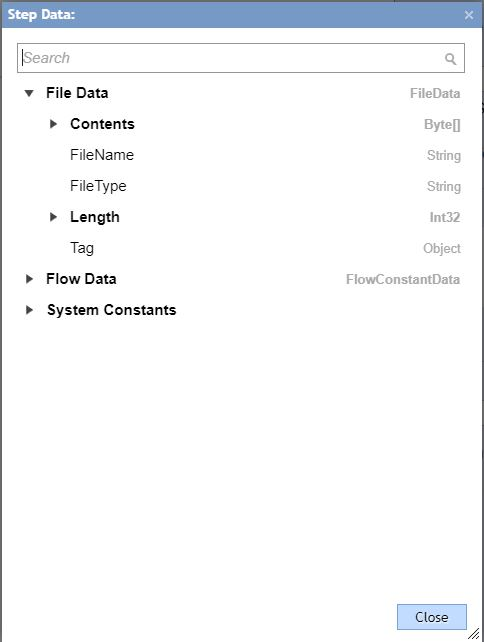• Version 6
• Updated on 04 Nov 2019
• Print
• Dark
Light

• Print
• Dark
Light

## Overview

A data type, or just "type," is a classification of data that tells the platform how you intend to use the data. The data type may be simple, such as Int32 (a 32-bit integer value) or Boolean (true or false value), or it may be a complex type such as a User Account or Database Structure. Simple types have only one value whereas complex types are structures that may have many values.
The following examples represent information that may be stored in a data type:

• The storage space that a variable of the type requires
• The maximum and minimum values that it can represent
• The members (methods, fields, events, and so on) that it contains
• The base type it inherits from
• The location where the memory for variables will be allocated at run time
• The kinds of operations that are permitted

## Data Types in Decisions

In Decisions, every variable (and every process that evaluates to a value) has a data type. Each step in the Flow Designer specifies a type for its input and output values. By default, Decisions defines a set of built-in alphanumeric types as well as more complex types that represent a wide variety of logical constructs. Decisions also lets you create custom data types, or "user-defined types." A typical flow will use some native types as well as one or more user-defined types.
When working with data in the Flow Designer, always consider the data type. For example, the figure below shows a String data type being used in a basic math function. Since String data types can contain letters or other non-numerical characters, the mapping editor shows an error. (Even if the value of the String data type is numerical it would still not work; the math function requires a numerical data type like Int32 or Decimal.)## Most Common Data Types in Decisions

For math calculations, use Integral and Floating Point Types as follows:

Integral Types

Type Range Size
SByte -128 to 127 Signed 8-bit integer
Byte 0 to 255 Unsigned 8-bit integer
Char U+0000 to U+ffff Unicode 16-bit character
Int16 -32,768 to 32,767 Signed 16-bit integer
Int32 -2,147,483,648 to 2,147,483,647 Signed 32-bit integer
Int64 -9,223,372,036,854,775,808 to 9,223,372,036,854,775,807 Signed 64-bit integer
UInt64 0 to 18,446,744,073,709,551,615 Unsigned 64-bit integer

Int32 Type is the most commonly used in Decisions for arithmetical calculations.

Floating Point Types

Type Approximate Range Precision
Single ±1.5e−45 to ±3.4e38 7 digits
Double ±5.0e−324 to ±1.7e308 15-16 digits
Decimal (-7.9 x 1028to 7.9 x 1028) / (100 to 28) 28-29 digits

Single and Double are floating binary point types. In other words, they represent a number like this:
10001.10010110011
The binary number and the location of the binary point are both encoded within the value.
Decimal is a floating decimal point type. In other words, they represent a number like this:
12345.65789

Again, the number and the location of the decimal point are both encoded within the value – that's what makes decimal still a floating-point type instead of a fixed point type.
For non-repeating decimal fractions and whole numbers, the most common data type is Decimal. This is usually suitable for any concepts invented by humans, such as financial values or product ratings.
For values that are either imprecise or immense (such as is found in artifacts of nature or which cannot be precisely measured), float/double data types are more appropriate. Scientific data is often represented in this form. With these data types, the original values are not "decimally accurate" to start with, so it is not important for the expected results to maintain the decimal accuracy. Floating binary point types are much faster to work with than decimals.

String - represents alphanumeric text as a series of Unicode characters. String Type is used for all text data manipulations.

TimeSpan - represents a time interval. The structure of this type is as follows:DateTime - represents an instant in time, typically expressed as a date and time of day.Boolean - represents a Boolean (true or false) value. Used in logical evaluations like ‘Yes’ or ‘No’.

FileData – represents File in Decisions. Contains following members: Content (Byte Array), FileName (String), FileType (String), Length (Int32), Tag (Object).